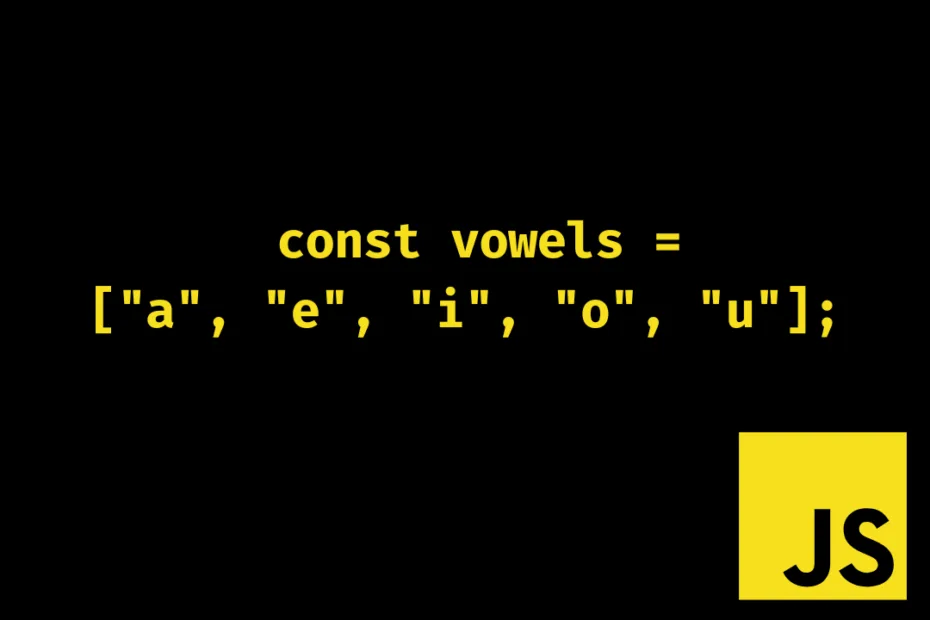# Count Vowels in a String with JavaScript

Problem Statement: For any given string write JavaScript code to console log the count of vowels with separate counts for each vowel occurrence. e.g if enter the string “apple” the output should be vowel count:: a:1, e:1, i:0, o:0, u:0. Let’s try to do this in a single iteration i.e. with Linear time complexity: O(n).

## Using for loop and switch case

Below we also used the Addition Assignment Operator (+=) which adds both left and right operands and assigns the result to the left operand.

``````// Using Addition Assignment Operator (+=)
a = a + b;
// Can also be written as
a += b;``````

First, we initialize an object to keep track of the count for each vowel occurrence and then we need to convert the string to either lowercase/uppercase for consistency.

``````// JavaScript code to count vowels in a string

const getVowelCount = (inputString) => {
const string = inputString.toLowerCase();
const vowelCount = { a: 0, e: 0, i: 0, o: 0, u: 0 };

for (let i = 0; i < string.length; i++) {
const character = string[i];
switch (character) {
case "a":
vowelCount.a += 1;
break;
case "e":
vowelCount.e += 1;
break;
case "i":
vowelCount.i += 1;
break;
case "o":
vowelCount.o += 1;
break;
case "u":
vowelCount.u += 1;
break;
}
}

const { a, e, i, o, u } = vowelCount;
const result = `Vowel Count:: a:\${a}, e:\${e}, i:\${i}, o:\${o}, u:\${u}.`;
return result;
};

console.log(getVowelCount("Oppenheimer"));
// Output > Vowel Count:: a:0, e:3, i:1, o:1, u:0.``````

## Vowel count with for of loop and error handling

We have used a for…of loop as the string is an iterable object and without error handling the above code can easily break just by passing null/undefined hence, we have added an error handling block that seems to handle most cases and if you find more edge cases drop a comment and we’ll fix that.

``````const getVowelCount = (inputString) => {
if (typeof inputString !== "string") {
return "Invalid String";
}

const vowelCount = { a: 0, e: 0, i: 0, o: 0, u: 0 };

for (let character of inputString.toLowerCase()) {
switch (character) {
case "a":
vowelCount.a += 1;
break;
case "e":
vowelCount.e += 1;
break;
case "i":
vowelCount.i += 1;
break;
case "o":
vowelCount.o += 1;
break;
case "u":
vowelCount.u += 1;
break;
}
}

const { a, e, i, o, u } = vowelCount;
const result = `Vowel Count:: a:\${a}, e:\${e}, i:\${i}, o:\${o}, u:\${u}.`;
return result;
};

console.log(getVowelCount("Barbie"));
// Output > Vowel Count:: a:1, e:1, i:1, o:0, u:0.

console.log(getVowelCount(undefined));
// Output > Invalid String``````

## Vowel count in a string with the array.includes()

The array.includes() checks if the given value is present in the array and returns either true or false. We have also added a total property to track the total no.of vowels in the string.

``````// JS code to get total and individual vowel count in a string

const getVowelCount = (inputString) => {
if (typeof inputString !== "string") {
return "Invalid String";
}

const vowels = ["a", "e", "i", "o", "u"];
const vowelCount = { a: 0, e: 0, i: 0, o: 0, u: 0, total:0 };

for (let character of inputString.toLowerCase()) {
if (vowels.includes(character)) {
vowelCount[character] += 1;
vowelCount.total += 1;
}
}

const { a, e, i, o, u, total } = vowelCount;
const result = `Vowel Count:: a:\${a}, e:\${e}, i:\${i}, o:\${o}, u:\${u}, total:\${total}.`;
return result;
};

console.log(getVowelCount("Barbenheimer"));
// Output > Vowel Count:: a:1, e:3, i:1, o:0, u:0, total:5.``````

## JS Regex code to count vowels in a string

In case you were looking for JavaScript code to simply count the total number of vowels in a string here’s a one-liner function using regex.

``````const getVowelCount = (string) => string.match(/[aeiou]/gi).length;
console.log(getVowelCount("Apple"));
// Output> 2``````

## Conclusion

Out of curiosity, I wanted to test the execution time for all the above codes and I tested them using performance.now() but all of them turned out almost the same. I’m still curious that there are more ways to do this in JavaScript in case you find something drops a comment. Good day!